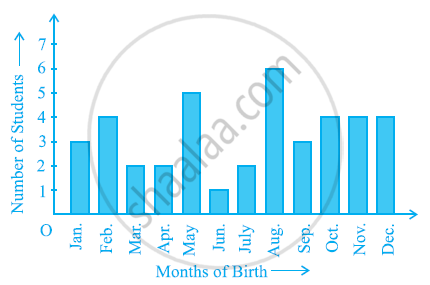# In a Particular Section of Class Ix, 40 Students Were Asked About the Months of Their Birth and the Following Graph Was Prepared for the Data So Obtained - Mathematics

In a particular section of Class IX, 40 students were asked about the months of their birth and the following graph was prepared for the data so obtained:-Find the probability that a student of the class was born in August.

#### Solution

Number of students born in the month of August = 6

Total number of students = 40

"P(Students born in the month of August) "="Number of students born in August"/"Total number of students"=6/40=3/20

Concept: Probability - an Experimental Approach
Is there an error in this question or solution?

#### APPEARS IN

NCERT Class 9 Maths
Chapter 15 Probability
Exercise 15.1 | Q 3 | Page 284